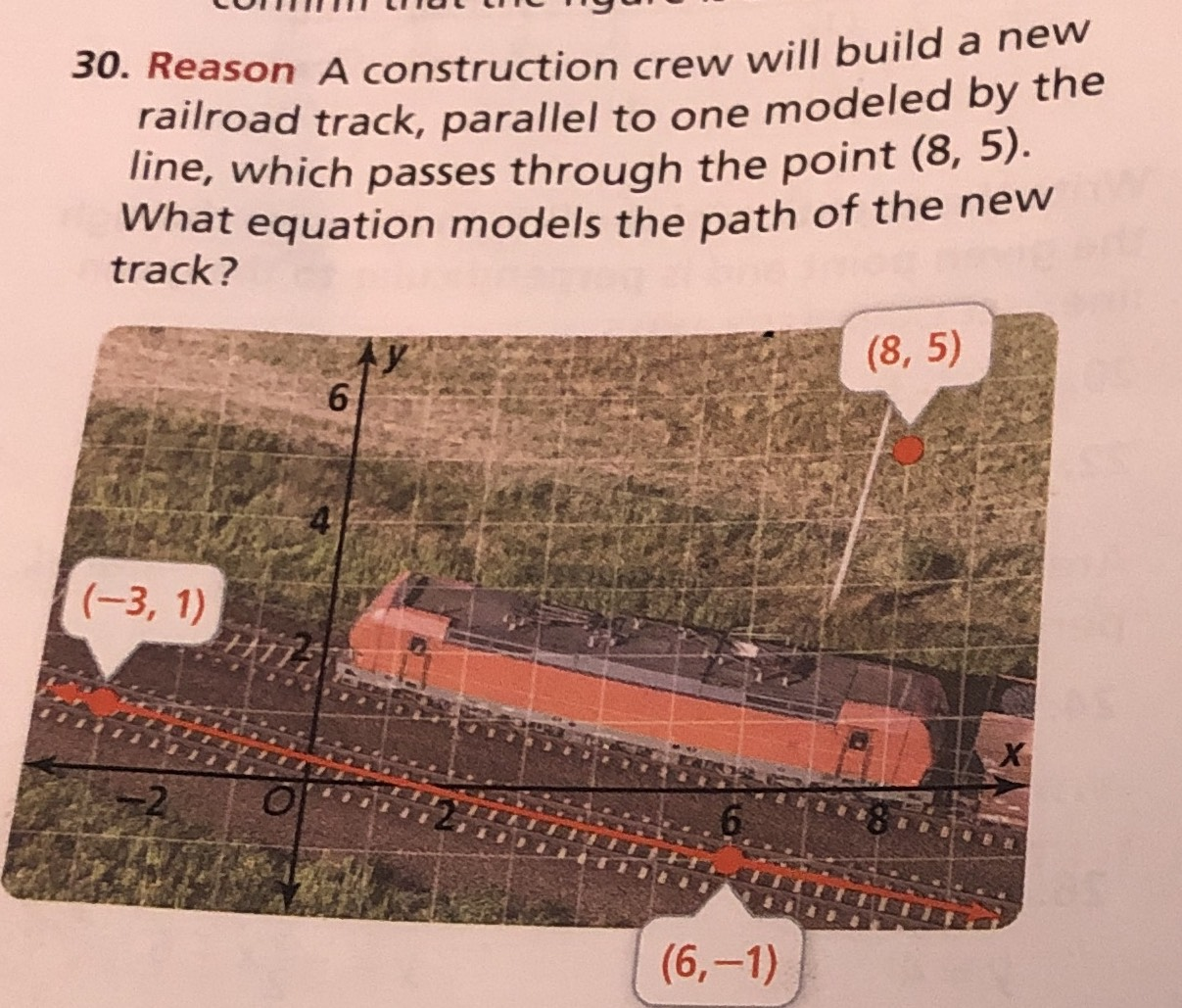### ¿Todavía tienes preguntas de matemáticas?

Pregunte a nuestros tutores expertos
Algebra
PreguntaA construction crew will build a new railroad track, parallel to one modeled by the line, which passes through the point $$( 8,5 )$$ . What equation models the path of the new track?

$$k= -\frac{2}{9},y= -\frac{2}{9}x+ \frac{61}{9}$$